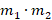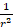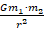# Newton's law of Universal Gravitation

Introduction

We are done with newton's third law now we are moving to newton's law  of gravitation. Have you asked yourself why do object fall down on earth not floating?. Sir Isaac Newton prove a theory that in every object on earth the is force of gravity that pulls object downward that is why people and objects are not floating.

Newton's law of universal gravitation is usually stated as that every particle attracts every other particle in the universe with a force that is directly proportional to the product of their masses and inversely proportional to the square of the distance between their centers.F αF =• SI units of m is Kg
• SI units of r is meter(m)
• Constant G=1.167×10-11

please watch the video which explain universal gravitation newton's law

For this task, you are required to find the gravitational force. Please read carefully and take notes of SI units. Round off to two decimal places.

1.Consider a man of mass 80 kg standing 10 m from a woman with a mass of 65 kg. Calculate the gravitational force between them.

2.A satellite orbits the earth 12 km above its surface. The mass of the satellite is 8.2×104 kg and the mass of the earth is 6×1024 kg. The radius of the earth is 6.4×106 m

3.Calculate the force between masses 100 g and 50 g if the distance between their centres is 67mm.

Allow the resources give to you to guide you on finding gravitational force

Process

• Write the data meaning you list what you are given.
• Check what what you are required to find.
• Check the SI units if mass is in grams you convert to Kg and distance in km/mm convert to metres(m).
• Remember the valued of G does not change
• Substitute  on formula.
• Round off to two decimal places.
• Write SI units on final answer.

Resources

Evaluation

These are marks for each solution

 1 4 2 7 3 6

1 mark for formula

1 mark for converting SI units

1 mark substitution

1 mark SI units

1 mark final answer rounded to two decimal places

Conclusion

The Universal gravitational newton will be on the test that will be writing next week. Please make sure you understand the videos and find other resources for more information.

Teacher Page

Zintle Vokwe

217232124